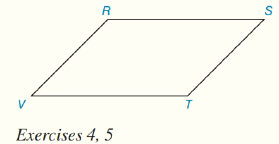Chapter 4.CT, Problem 5CTElementary Geometry For College St...

7th Edition
Alexander + 2 others
ISBN: 9781337614085

Solutions

Chapter
SectionElementary Geometry For College St...

7th Edition
Alexander + 2 others
ISBN: 9781337614085
Textbook Problem

In ▱ R S T V , V T = 3 x − 1 , T S = 2 x + 1 ,  and  R S = 4 ( x − 2 ) . Find the value of x.To determine

To Find:

The value of x.

Explanation

Given:

Given parallelogram is RSTV,

.

Calculation:

From the property opposite sides in a parallelogram have same length.

Now, from the figure,

RS¯=VT¯4

Still sussing out bartleby?

Check out a sample textbook solution.

See a sample solution

The Solution to Your Study Problems

Bartleby provides explanations to thousands of textbook problems written by our experts, many with advanced degrees!

Get Started

Find the limit or show that it does not exist. limx(4x2+3x+2x)

Single Variable Calculus: Early Transcendentals, Volume I

In Exercises 2124, factor each expression completely. 23. 16 x2

Applied Calculus for the Managerial, Life, and Social Sciences: A Brief Approach

Graph the following points: P(4,2),Q(2,4),R(1,3),S(4,2),T(2,1),U(2,0),V(4,4)

Finite Mathematics and Applied Calculus (MindTap Course List)